# Engineering Mechanics - Planar Kinematics of a Rigid Body (PKRB)

### Exercise :: Planar Kinematics of a Rigid Body (PKRB) - General Questions

• Planar Kinematics of a Rigid Body (PKRB) - General Questions
16.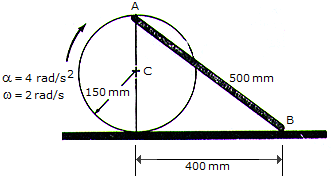The disk rolls without slipping such that it has an angular acceleration of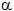= 4 rad/s2 and angular velocity of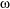= 2 rad/s at the instant shown. Determine the accelerations of points A and B on the link and the link's angular acceleration at this instant. Assume point A lies on the periphery of the disk, 150 mm from C.

 A. aA = (1.200i - 0.600j) m/s2, aB = 1.650i m/s2, "AB = 1.500 rad/s2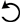B. aA = (1.200i - 0.600j) m/s2, aB = 1.650i m/s2, "AB = 1.500 rad/s2C. aA = (1.200i - 2.40j) m/s2, aB = 3.00i m/s2, "AB = 6.00 rad/s2D. aA = (1.200i - 1.200j) m/s2, aB = 2.10i m/s2, "AB = 3.00 rad/s2Explanation:

No answer description available for this question. Let us discuss.

17.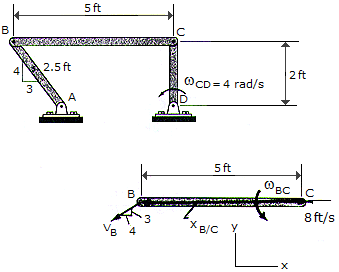Knowing the angular velocity of link CD isCD = 4 rad/s, determine the angular velocities of links BC and AB at the instant shown.

 A.AB = 4.00 rad/s TBC = 1.200 rad/s B.AB = 2.65 rad/s TBC = 1.985 rad/s C.AB = 4.00 rad/s TBC = 1.200 rad/s D.AB = 1.600 rad/s TBC = 2.29 rad/s

Explanation:

No answer description available for this question. Let us discuss.

18.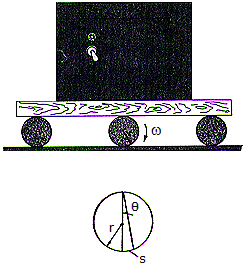The safe is transported on a platform which rests on rollers, each having a radius r. If the rollers do not slip, determine their angular velocityif the safe moves forward with a velocity v.

 A.= v/(2r) B.= v/r C.= 3v/r D.= 2v/r

Explanation:

No answer description available for this question. Let us discuss.

19.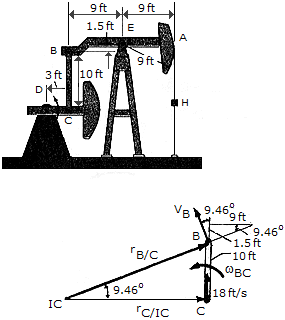The oil pumping unit consists of a walking beam AB, connecting rod BC, and crank CD. If the crank rotates at a constant rate of 6 rad/s, determine the speed of the rod hanger H at the instant shown.

 A. vH = 17.76 ft/s B. vH = 16.20 ft/s C. vH = 18.00 ft/s D. vH = 16.42 ft/s

Explanation:

No answer description available for this question. Let us discuss.

20.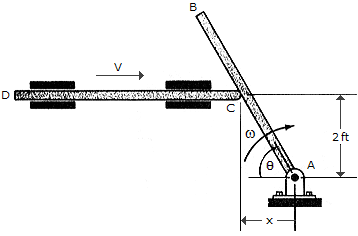Rod CD presses against AB, giving it an angular velocity. If the angular velocity of AB is maintained at= 5 rad/s, determine the required speed v of CD for any angleof rod AB.

 A. vCD = 10 csc2B. vCD = -10 csc2C. vCD = -10 sinD. vCD = 10 sin